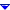Determine your best and worst investment returns
Your best and worst performing investments are determined by their Return on Investment (ROI) ( Latest 12-months’ net income divided by invested capital (long-term debt plus common stock equity and preferred equity). The invested capital can be the figure at the end of the quarter or the average over the year. ) percentage for each time period.

To see a list of your best and worst performing investments:
1. Go to the Portfolio Manager.
2. In the left pane, under Common tasks, click Review portfolio.
3. Scroll down to Performance.

Following are the formulas used for determining the ROI:

• Over the past month: [(current value) - (value 4 weeks ago) + (income)] / (value 4 weeks ago)
• So far this year: [(current value) - (value last January 1) + (income)] / (value last January 1)
• Over the past 12 months: [(current value) - (value 12 months ago) + (income)] / (value 12 months ago)
• Over the entire history of your portfolio: [(current value) - (cost basis) + (income)] / (cost basis)
Related topic

Print a chart